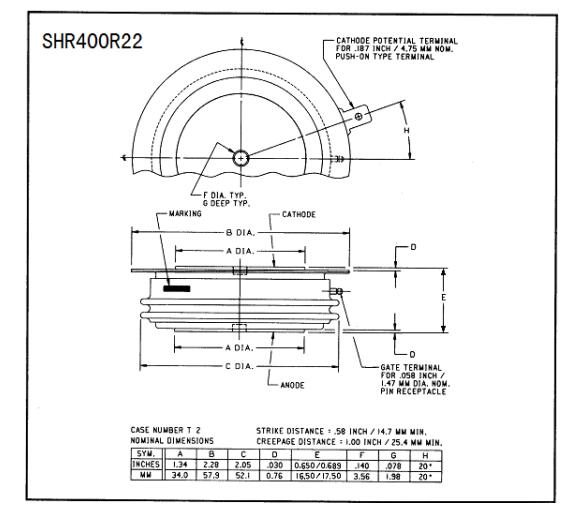# 1300v fast switching reverse conducting thyristor rct

• YZPST-SHR400R22

YZPST-SHR400R22 Fast Switching Reverse-conducting Thyristor

RCT FOR INVERTER AND CHOPPER APPLICATIONS

Features:

All Diffused Structure

Interdigitated Amplifying Gate Configuration

Blocking capabilty up to 1300 volts

Guaranteed Maximum Turn-Off Time

High dV/dt Capability

Pressure Assembled Device

"

 Repetitive peak off state leakage IDRM 35 mA Critical rate of voltage rise dV/dt 1000 V/msec

Conducting - on state

 Parameter Symbol Min. Max. Typ Units Conditions RMS value of on-state current ITRMS 630 A Nominal value Average on-state current IT(AV) 400 A Continuous single-phase,half sine wave,180°conduction Peak one cycle surge on-state current(non repetitive) ITSM 7200 A 50Hz, sinusoidal wave-shape, 180o conduction, Tj = 115 oC I2t Limit Value I2t 200x103(On-Current) A2s 31x103(Reverse Current) Peak on-state voltage VTM 3.0 V ITM =1250A; Tj = 25 oC Critical rate of rise of on-statecurrent di/dt 100 A/ms VD=1/2VDRM,ITM=800A f=60HZIGM=1.5A,diG/dt=1.0A/us,Tj=115℃ Average reverse current IR(AV) 150 A Continuous single-phase,half sine wave,180°conduction RMS reverse currrnt IR(RMS) 235 A Peak reverse voltage VRM 2.5 V IRM=500A, Tj = 25 oC Peak one cycle surge  reverse current(non repetitive) IRSM 2500 A 50Hz, sinusoidal wave-shape, 180o conduction, Tj = 115 oC Critical rate of rise of commutating off-state voltage (dv/dt)C 200 A/ms ITM=2000A,tw=60us,IRM=1000A, ,VDM=1/2VDRM,Pulse width 60ms ,Tj=115℃,

Gating

 Parameter Symbol Min. Max. Typ. Units Conditions Peak gate power dissipation PGM 20 W tp = 40 us Average gate power dissipation PG(AV) 4 W Peak gate current IGM 4 A Gate current required to trigger all units IGT 200 mA VD = 6 V;RL = 6 ohms;TC = 25 oC Holding current IH 500 Tj = 25 oC ;RL = 6 ohms Gate voltage required to trigger all units VGT 3.0 V VD = 6 V;RL = 6 ohms;TC = 25oC Peak non- trigger voltage VGD 0.15 V Tj = 115 oC;VD=1/2VDRM

Dynamic

 Parameter Symbol Min Max. Typ. Units Conditions Turn-off time tq 40 ms ITM = 400 A; di1/dt = -50A/ms; di2/dt=50A/us,IRM=10A; dV/dt(c) =200 V/ms VDR=650V Tj = 115 oC;tw=60us

CASE OUTLINE AND DIMENSIONS.             Reverse-conducting Thyristor"